# Introduction

This knowledge base article expands upon the article Optimizing for a Target Spatial Distribution to demonstrate how to use FRED's Bitmap source type to generate an arbitrary normalized target irradiance pattern to use in subsequent optimizations. A modified optimization merit function aberration based on a user-defined script is also provided.

## Target Bitmap Creation

To create a target spatial distribution in FRED simply requires a bitmap which can be made in any drawing software (such as MS Paint). For a simple ideal rectangular uniform irradiance target the bitmap may look something like this:## Target ARN Creation

Once the desired bitmap target is made, we can use the Source Bitmap option to trace rays based on the bitmap values. In the case of the above bitmap rays are generated from the white pixels and no rays are generated from the black pixels.

The bitmap source should be set up to use the bitmap (or jpeg) file previously defined with a suitable width and a small angular range of emission such that the generated rays are essentially collimated: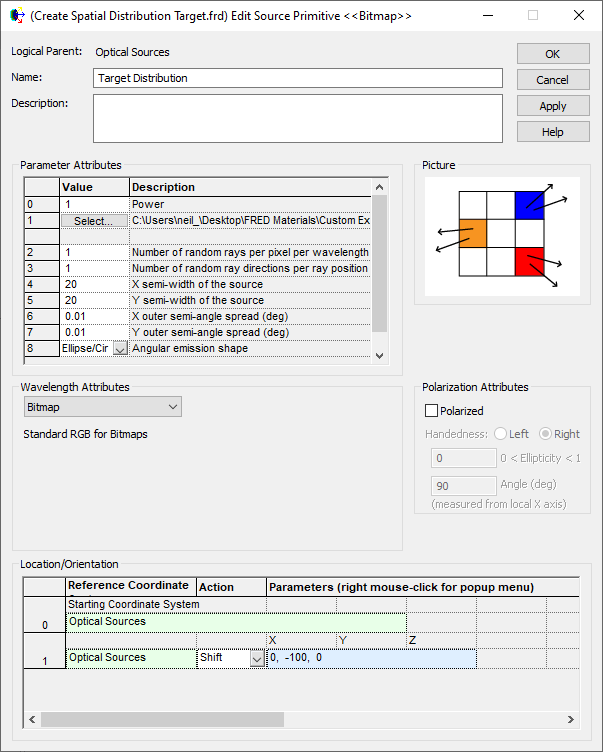After this source we can place a geometry surface (with an attached analysis surface) very close to the source to capture the distribution: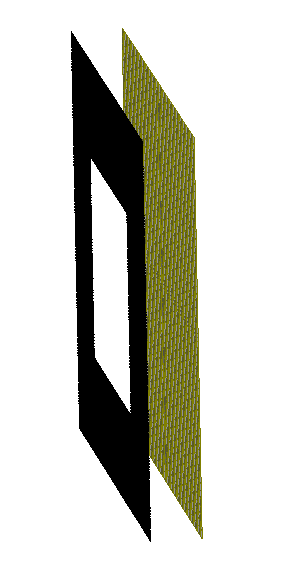Tracing the rays and running an Irradiance Calculation with the option checked to create the ARN: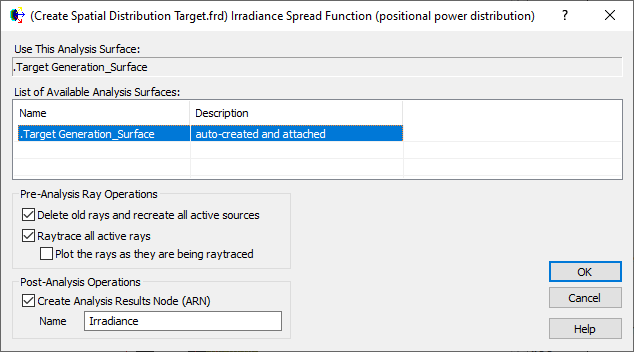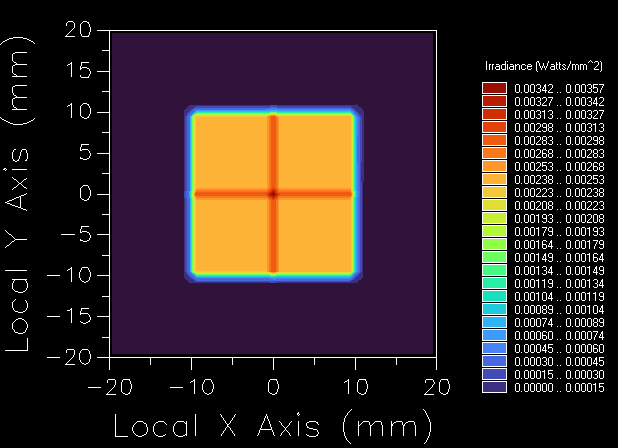The resulting irradiance has two bright bands due to the pixel sampling. We can remove these by choosing the 'Smooth/Modify Data' option from the r-click menu on the chart and applying a suitable ceiling to the data: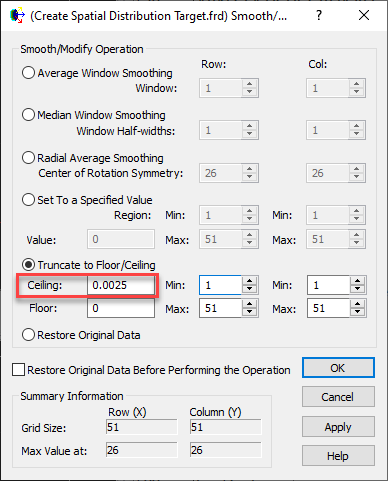And then we can normalize the data to a peak value of unity from the right-click chart menu to 'Scale Data':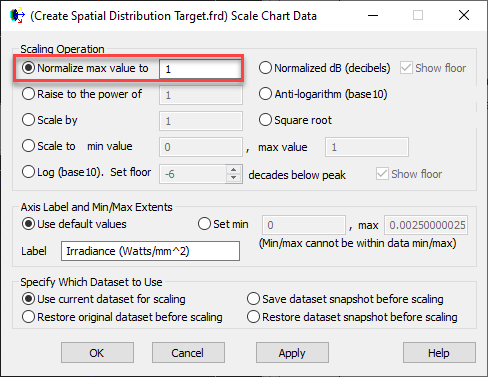And this gives the final normalized target distribution: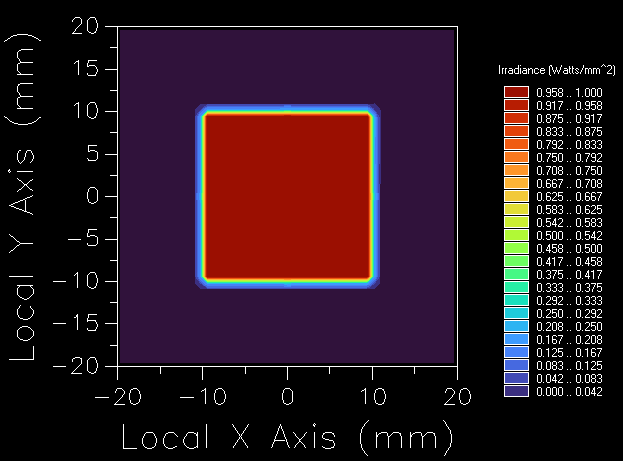From this chart we can right-click and choose the option to 'Export Data' to a .dat file.

The resulting .dat file then needs to be modified to add the header data required to allow it to be read back in as an Irradiance ARN. Edit the .dat file to add the following above the existing pixel data, noting that the AXIS_MIN, _MAX and _DIM values need to be modified set to match the analysis surface in terms of its size and number of pixels:

```FRED_DATA_FILE
FILETYPE= Grid2D
DATATYPE= Double
VERSION= 2
DATAUNITS= "Watts/mm^2"
POWERUNITS= "Watts"
DATETIME= "Thursday, January 21, 2021 17:29:28 (GMT Standard Time)"
ORIGIN_POSITION= 0 -100 1
BINARY= FALSE
HOLE_VALUE= 1e+308
!------------------------------------------------------------------------
A_AXIS_MIN= -20
A_AXIS_MAX= 20
A_AXIS_DIM= 21
A_AXIS_LABEL= "Local X Axis"
A_AXIS_TYPE= Spatial
A_AXIS_UNITS= mm
A_AXIS_DIR= 1 0 0
!------------------------------------------------------------------------
B_AXIS_MIN= -20
B_AXIS_MAX= 20
B_AXIS_DIM= 21
B_AXIS_LABEL= "Local Y Axis"
B_AXIS_TYPE= Spatial
B_AXIS_UNITS= mm
B_AXIS_DIR= 0 1 0
!------------------------------------------------------------------------
BeginData
```

With these steps completed - this file can be read back into any FRED model as a target ARN by using the option to 'Create New ARN from a File...':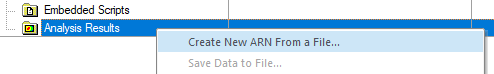## Modified Optimization Aberration Script

The merit function aberration being used is a User-defined script, since there currently exists no default method for importing a target distribution into the optimizer. The script used to implement the aberration to target a distribution defined by an ARN is provided below with comments.

```Sub EvalMzrAber( ByVal g_ana&, ByRef g_aber#, ByRef g_success As Boolean )

Dim arnStats As T_ARN_2D_CELL_STATS
Dim TargetARN As Long, ActualARN As Long, numRays As Long, ii As Long, jj As Long
Dim ActualData() As Double, TargetData() As Double, PeakActualPower As Double, Phi As Double
Dim success As Boolean

'find node number of the peak normalized target ARN - MUST BE CALLED "Irradiance Target"
TargetARN = ARNFindName( "Irradiance Target" )

'store the traced irradiance distribution in an ARN and renormalize the power to peak
numRays = IrradianceToARN( g_ana, "Actual ARN", ActualARN )

'Find peak value
ARNCompute2DCellStatistics ActualARN, arnStats
PeakActualPower = arnStats.MaxCellVal

'Loop over ActualData and normalize to peak
ARNGetDataAsDoubleArray ActualARN, ActualData()
For ii = 0 To UBound(ActualData,1)
For jj = 0 To UBound(ActualData,2)
ActualData(ii,jj) = ActualData(ii,jj)/PeakActualPower
Next jj
Next ii
ARNSetDataAsDoubleArray ActualARN, ActualData()

'difference the two ARN. (Actual - Target). Results are stored in ActualARN node.
ARNLinearCombination( 1, ActualARN, -1, TargetARN )

'ActualARN now contains (actual - target) and so we just need to loop over this and square the values.
'Phi is error value (actual - target) ^ 2
'want to return sum of the squares - so keep running tally of sum of Phi as we loop through.

Phi = 0

ARNGetDataAsDoubleArray ActualARN, ActualData()
For ii = 0 To UBound(ActualData,1)
For jj = 0 To UBound(ActualData,2)
'ActualData(ii,jj) = Abs( ActualData(ii,jj) )
Phi = Phi + (ActualData(ii,jj))^2
Next jj
Next ii

g_aber = Phi

'delete the ARN nodes (memory management)
success = ARNDelete( ActualARN )

g_success = PeakActualPower > 0

End Sub
```